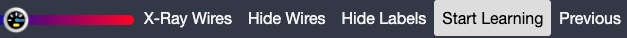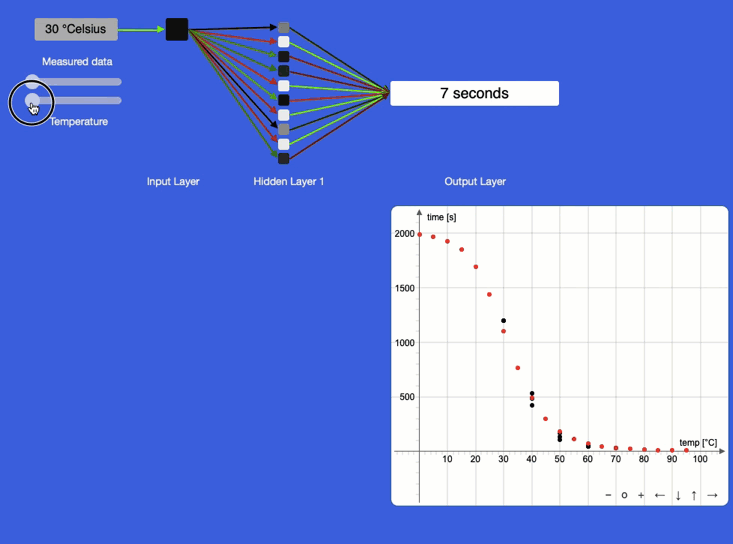top of page

# AI ScientistIn science, students conduct experiments during which they measure all sorts of things, such as time, temperature, force, etc. Students collect the data in a table, plot the data points in a cartesian plane and then try to find a curve that best fits the data. For this, they need to formulate a hypothesis. Is the relationship linear? Exponential? Logarithmic? Polynomial? Or perhaps a combination of some of them?

What if an AI could find the best curve and make predictions of what is happening between and outside our data points we have measured in the lab? For our scientist readers: Yes, we are talking about interpolation and extrapolation.

Artificial Neural Networks (ANNs) are essentially multi-dimensional optimisation engines. Let's see if they are up to the job. We start with the experiment to obtain data.

A science experiment

A student investigated the influence of temperature on the reaction rate of hydrogen peroxide and potassium sodium tartrate with the catalyst cobalt chloride. The student measured reaction times three times each for 30, 40, 50, 60, and 70 °C. The experiment didn't finish at 30°C, so the student entered the maximum elapsed time of 1200 seconds = 20 min. The student recorded the data in a table:

The student then charted the data.AI training data

The training data is easily obtained from the experiment. Internally the ANN works with numbers from 0 to 1, so we need to normalise our data. Some ANNs do this automatically for us, so let's take a quick look at how this works. First, we find the maximum values for temperature and time. From the table, that's 70°C and 1,500 seconds. We want a little bit of margin, and go with 100°C and 2,000 seconds.

We divide all our temperature values by 100 and our time values by 2,000. This leads us to the normalised data, which the AI can work with.

The learning process

Let's run the training process by clicking on the start learning button in the top right corner of our screen.The training data will be shown to the AI and it will make a series of repeated improvement attempts until the error of the network falls below a preset threshold. Sit back and observe how the red dots, representing the ANN's knowledge base, start moving towards the black dots (our lab data). The video below is a real-time recording. The seconds are a calculated output from the AI and not a progress indicator. The process is fast!We can now drag the temperature slider to set temperature values between and outside of our experimental data. You can see this as the red curve in the image below. This visualisation helps us to form a hypothesis and, if we want, test this further in the lab. In this particular case, the part of the curve for temperature values above 30°C looks a lot like an exponential function in the general form y=a*e^(-bx).If you want to try this yourself, head over here: You will need to create a free teacher account, which takes no more than 30 seconds. Student accounts can be purchased from the built-in shop for a reasonable amount of \$5.

Let us know what you think.

Until next time,

The Doctor.

Acknowledgement. We want to thank Esther Schulz, year 11 student from Kenmore State High School in Brisbane for conducting the experiment and for allowing us to use her data.

Tags: